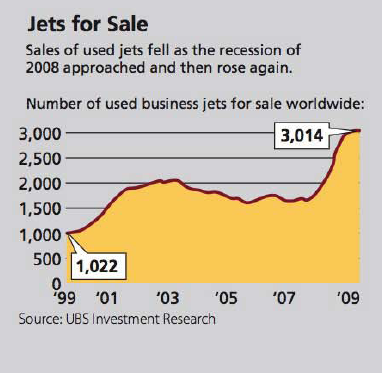Chapter 6, Problem 35AT### Contemporary Mathematics for Busin...

8th Edition
Robert Brechner + 1 other
ISBN: 9781305585447

#### Solutions

Chapter
Section### Contemporary Mathematics for Busin...

8th Edition
Robert Brechner + 1 other
ISBN: 9781305585447
Textbook Problem

# Solve the following word problems for the unknown. Round decimals to hundredths and percents to the nearest tenth when necessary.Last year Keystone’s corporate jet required $23,040 in maintenance and repairs.a. If this represents 32% of the total operating costs of the airplane, what was the total cost to fly the plane for the year?b. If the plane flew 300,000 miles last year, what is the cost per mile to operate the plane?c. Sky King Leasing offered a deal whereby it would operate the plane for Keystone for only$0.18 per mile. What is the percent decrease in operating expense per mile being offered by Sky King?d. In one ten-year period, the sales of used business jets rose and fell and then rose again. Use the chart “Jets for Sale” to calculate the rate of increase of jets available in 2009 compared with 1999. Round to the nearest whole percent.(a)

To determine

To calculate: The total cost to fly the plane for the year if maintenance and repair represents 32% of the total operating cost of the airplane and last year Keystone’s corporate jet required $23,040 in it. Explanation Given Information: It is given that Keystone’s corporate jet required$23,040 in maintenance and repair and it represents 32% of the total operating cost of the airplane.

Formula used:

Base=PortionRate

Calculation:

Consider the value of rate is R=32% and the value of portion is P=$23,040. Here the total cost to fly the plane for the year is unknown therefore the missing value is base as base is the whole amount. The formula to compute the base is Base=PortionRate Transform percent or rate to a decimal value as, R=32 (b) To determine To calculate: The cost per mile to operate the plane, if the plane flew 300,000 miles last year and Keystone’s corporate jet required$23,040 in maintenance and repair last year.

(c)

To determine

To calculate: The percent decrease offered by Sky King in operating expense per mile such that it offered a deal according to which it operate the plane for Keystone for only \$0.18 per mile.

(d)

To determine

To calculate: The rate of increase of jets available in 2009 compared with 1999 by using the chart and also round it to nearest whole percent.

### Still sussing out bartleby?

Check out a sample textbook solution.

See a sample solution

#### The Solution to Your Study Problems

Bartleby provides explanations to thousands of textbook problems written by our experts, many with advanced degrees!

Get Started

#### In Exercises 516, evaluate the given quantity. log218

Finite Mathematics and Applied Calculus (MindTap Course List)

#### In problems 45-62, perform the indicated operations and simplify. 60.

Mathematical Applications for the Management, Life, and Social Sciences

#### 27r63s2t4

Applied Calculus for the Managerial, Life, and Social Sciences: A Brief Approach

#### Draw a scatterplot for which r = 1.

Introduction To Statistics And Data Analysis